## Understanding Neural Networks. From neuron to RNN, CNN, and Deep LearningArticle originally posted on Data Science Central. Visit Data Science Central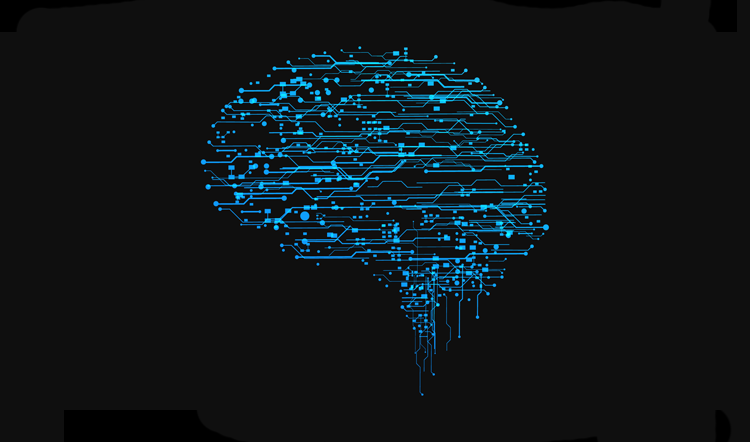Neural Networks is one of the most popular machine learning algorithms at present. It has been decisively proven over time that neural networks outperform other algorithms in accuracy and speed. With various variants like CNN (Convolutional Neural Networks), RNN(Recurrent Neural Networks), AutoEncoders, Deep Learning etc. neural networks are slowly becoming for data scientists or machine learning practitioners what linear regression was one for statisticians. It is thus imperative to have a fundamental understanding of what a Neural Network is, how it is made up and what is its reach and limitations. This post is an attempt to explain a neural network starting from its most basic building block a neuron, and later delving into its most popular variations like CNN, RNN etc.

What is a Neuron?

As the name suggests, neural networks were inspired by the neural architecture of a human brain, and like in a human brain the basic building block is called a Neuron. Its functionality is similar to a human neuron, i.e. it takes in some inputs and fires an output. In purely mathematical terms, a neuron in the machine learning world is a placeholder for a mathematical function, and its only job is to provide an output by applying the function on the inputs provided.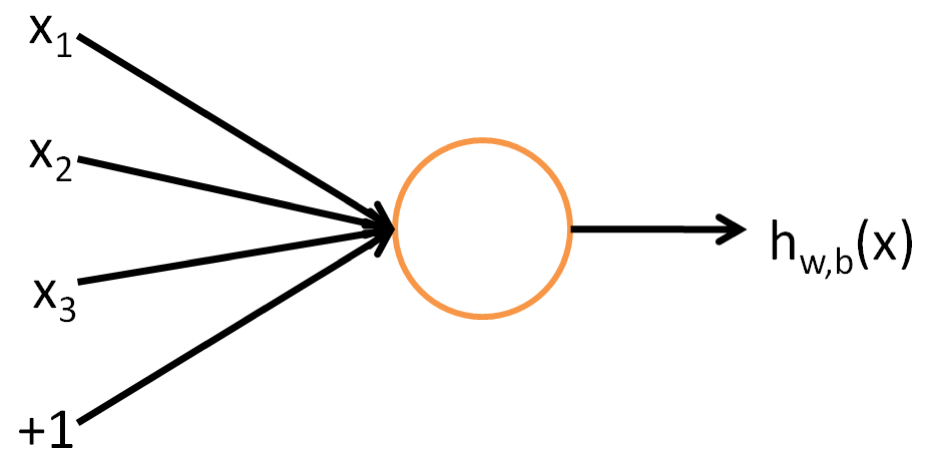The function used in a neuron is generally termed as an activation function. There have been 5 major activation functions tried to date, step, sigmoid, tanh, and ReLU. Each of these is described in detail below.

ACTIVATION FUNCTIONS

Step function

A step function is defined as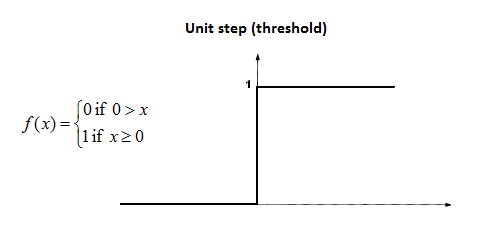Where the output is 1 if the value of x is greater than equal to zero and 0 if the value of x is less than zero. As one can see a step function is non-differentiable at zero. At present, a neural network uses back propagation method along with gradient descent to calculate weights of different layers. Since the step function is non-differentiable at zero hence it is not able to make progress with the gradient descent approach and fails in the task of updating the weights.

To overcome, this problem sigmoid functions were introduced instead of the step function.

Sigmoid Function

A sigmoid function or logistic function is defined mathematically as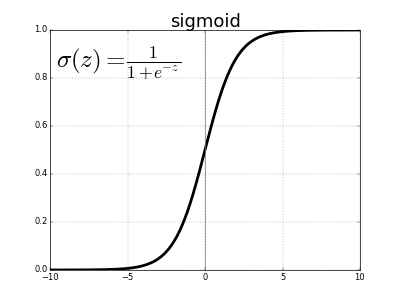The value of the function tends to zero when z or independent variable tends to negative infinity and tends to 1 when z tends to infinity. It needs to be kept in mind that this function represents an approximation of the behavior of the dependent variable and is an assumption. Now the question arises as to why we use the sigmoid function as one of the approximation functions. There are certain simple reasons for this.

1. It captures non-linearity in the data. Albeit in an approximated form, but the concept of non-linearity is essential for accurate modeling.

2. The sigmoid function is differentiable throughout and hence can be used with gradient descent and backpropagation approaches for calculating weights of different layers

3. The assumption of a dependent variable to follow a sigmoid function inherently assumes a Gaussian distribution for the independent variable which is a general distribution we see for a lot of randomly occurring events and this is a good generic distribution to start with.

However, a sigmoid function also suffers from a problem of vanishing gradients. As can be seen from the picture a sigmoid function squashes it’s input into a very small output range [0,1] and has very steep gradients. Thus, there remain large regions of input space, where even a large change produces a very small change in the output. This is referred to as the problem of vanishing gradient. This problem increases with an increase in the number of layers and thus stagnates the learning of a neural network at a certain level.

Tanh Function

The tanh(z) function is a rescaled version of the sigmoid, and its output range is [ − 1,1] instead of [0,1].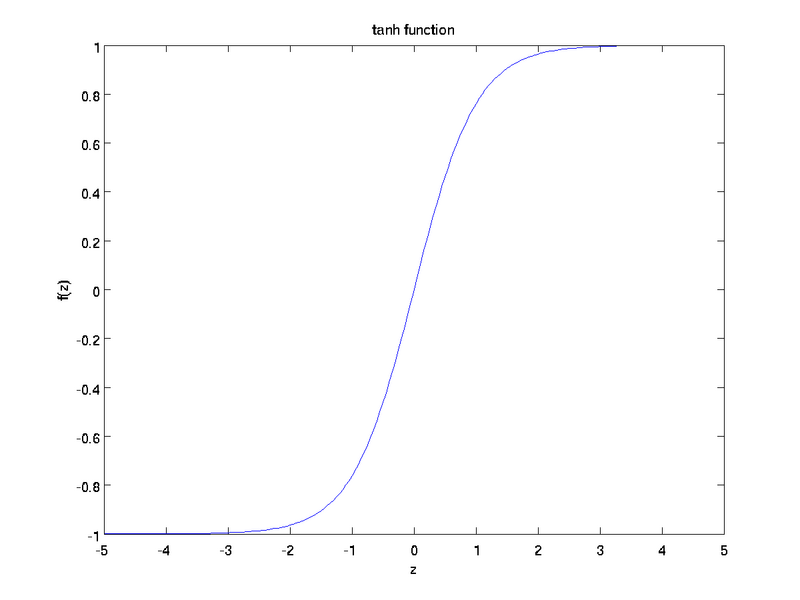The general reason for using a Tanh function in some places instead of the sigmoid function is because since data is centered around 0, the derivatives are higher. A higher gradient helps in a better learning rate. Below attached are plotted gradients of two functions tanh and sigmoid. 

For tanh function, for an input between [-1,1], we have derivative between [0.42, 1].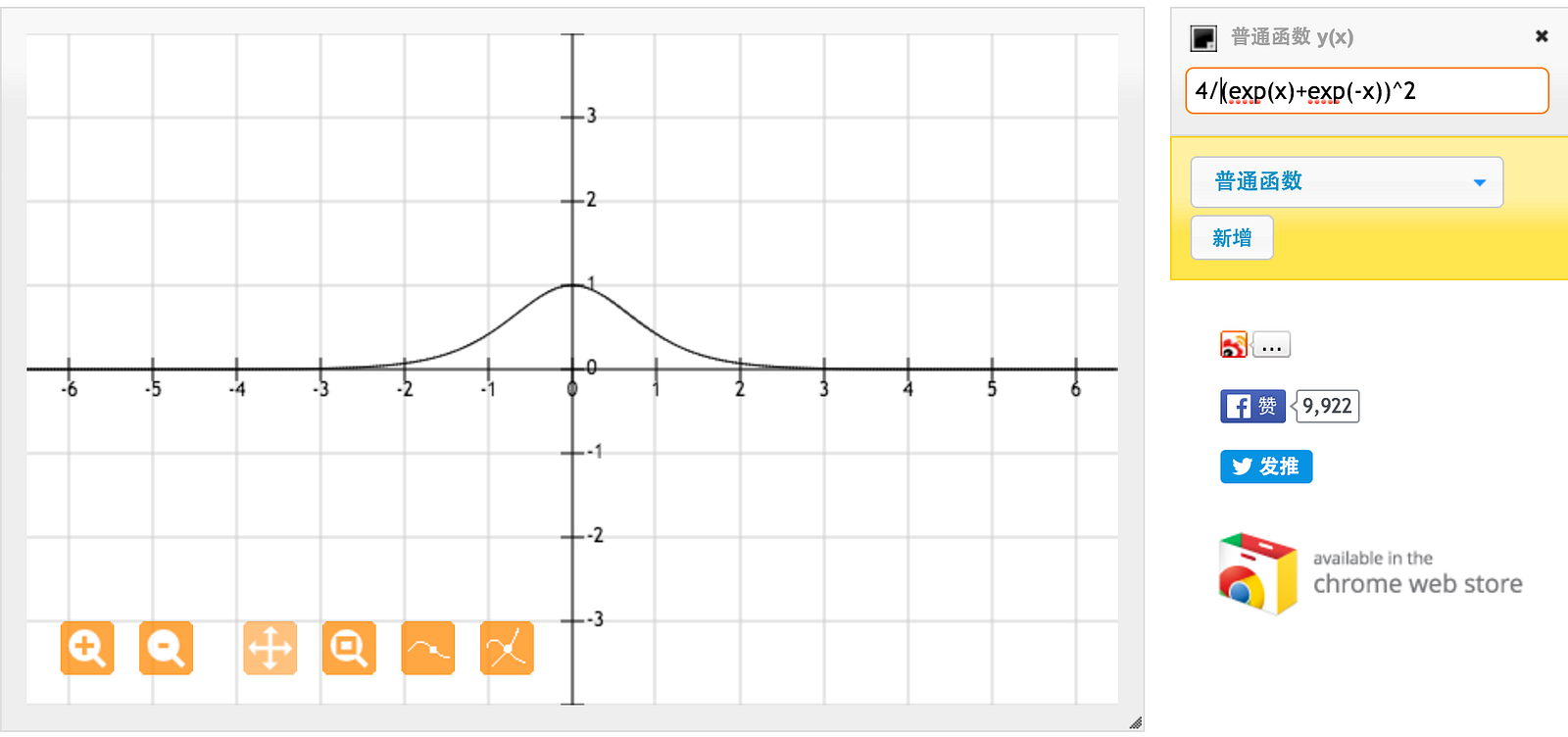For sigmoid function on the other hand, for input between [0,1], we have derivative between [0.20, 0.25]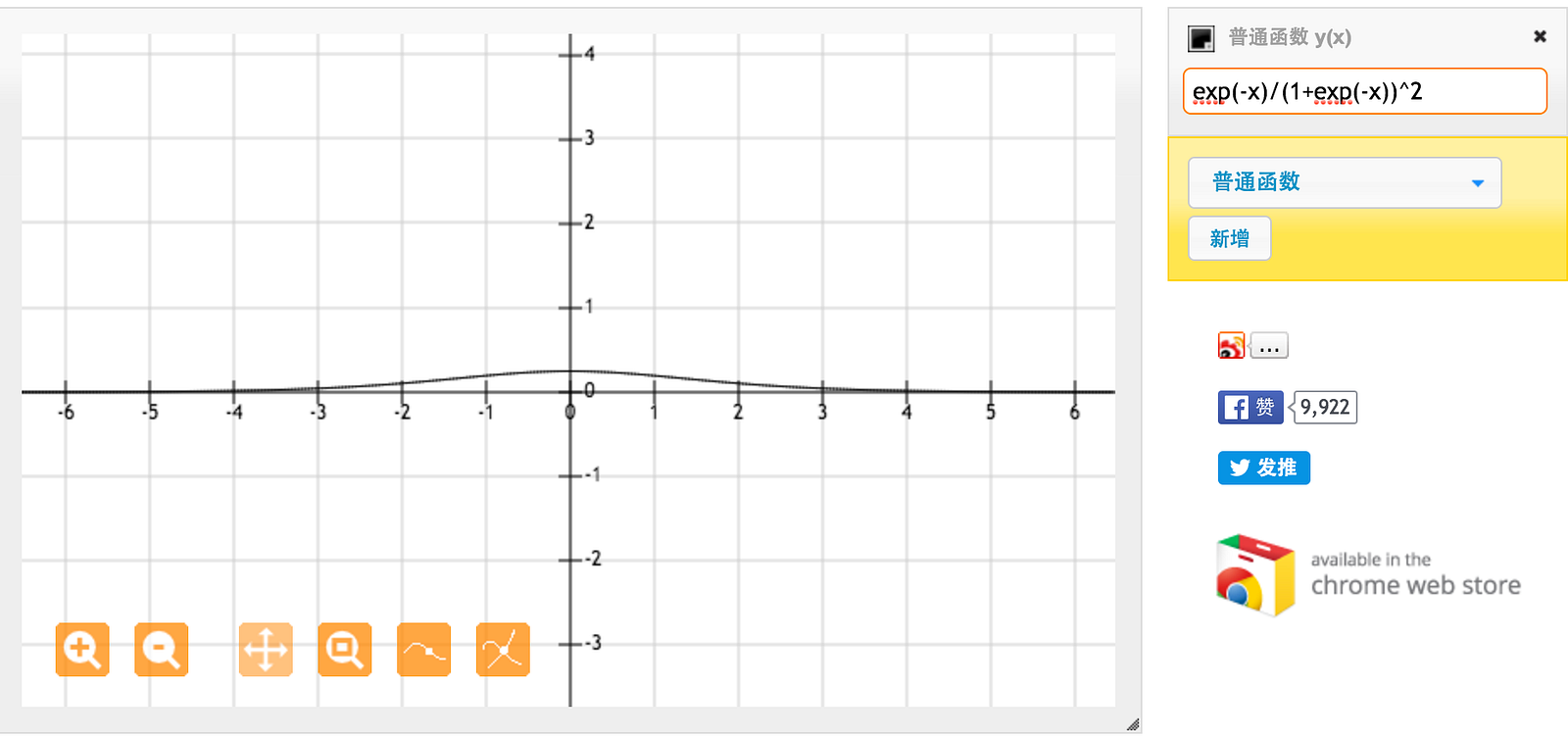As one can see from the pictures above a Tanh function has a higher range of derivative than a Sigmoid function and thus has a better learning rate. However, the problem of vanishing gradients still persists in Tanh function.

ReLU Function

The Rectified Linear Unit is the most commonly used activation function in deep learning models. The function returns 0 if it receives any negative input, but for any positive value x, it returns that value back. So, it can be written as f(x)=max (0, x).

Graphically it looks like this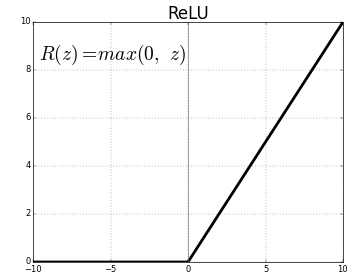The Leaky ReLU is one of the most well-known. It is the same as ReLU for positive numbers. But instead of being 0 for all negative values, it has a constant slope (less than 1.).

That slope is a parameter the user sets when building the model, and it is frequently called α. For example, if the user sets α=0.3, the activation function is.`f(x) = max (0.3*x, x)` This has the theoretical advantage that, by being influenced, by `x` at all values, it may make more complete use of the information contained in x.

There are other alternatives, but both practitioners and researchers have generally found an insufficient benefit to justify using anything other than ReLU. In general practice as well, ReLU has found to be performing better than sigmoid or tanh functions.

Neural Networks

Till now we have covered neuron and activation functions which together for the basic building blocks of any neural network. Now, we will dive in deeper into what is a Neural Network and different types of it. I would highly suggest people, to revisit neurons and activation functions if they have a doubt about it.

Before understanding a Neural Network, it is imperative to understand what is a layer in a Neural Network. A layer is nothing but a collection of neurons which take in an input and provide an output. Inputs to each of these neurons are processed through the activation functions assigned to the neurons. For example, here is a small neural network.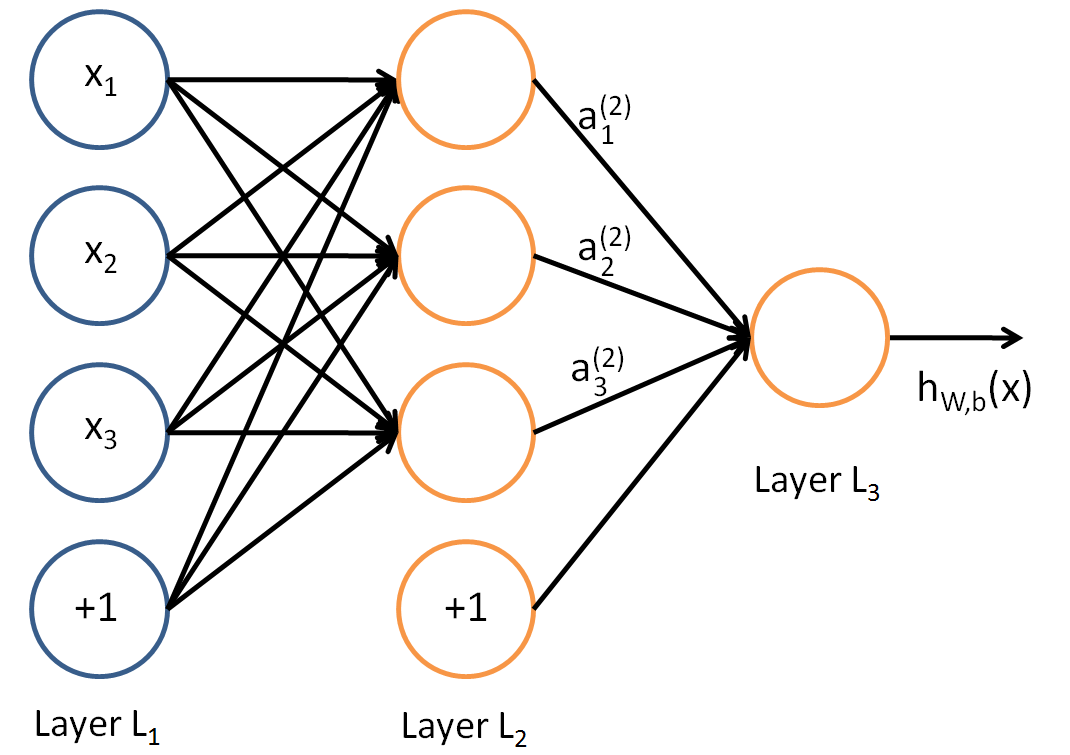The leftmost layer of the network is called the input layer, and the rightmost layer the output layer (which, in this example, has only one node). The middle layer of nodes is called the hidden layer because its values are not observed in the training set. We also say that our example neural network has 3 input units (not counting the bias unit), 3 hidden units, and 1 output unit 

Any neural network has 1 input and 1 output layer. The number of hidden layers, for instance, differ between different networks depending upon the complexity of the problem to be solved.

Another important point to note here is that each of the hidden layers can have a different activation function, for instance, hidden layer1 may use a sigmoid function and hidden layer2 may use a ReLU, followed by a Tanh in hidden layer3 all in the same neural network. Choice of the activation function to be used again depends on the problem in question and the type of data being used.

Now for a neural network to make accurate predictions each of these neurons learn certain weights at every layer. The algorithm through which they learn the weights is called back propagation, the details of which are beyond the scope of this post.

A neural network having more than one hidden layer is generally referred to as a Deep Neural Network.

Convolutional Neural Networks (CNN)

Convolutional Neural Networks (CNN) is one of the variants of neural networks used heavily in the field of Computer Vision. It derives its name from the type of hidden layers it consists of. The hidden layers of a CNN typically consist of convolutional layers, pooling layers, fully connected layers, and normalization layers. Here it simply means that instead of using the normal activation functions defined above, convolution and pooling functions are used as activation functions.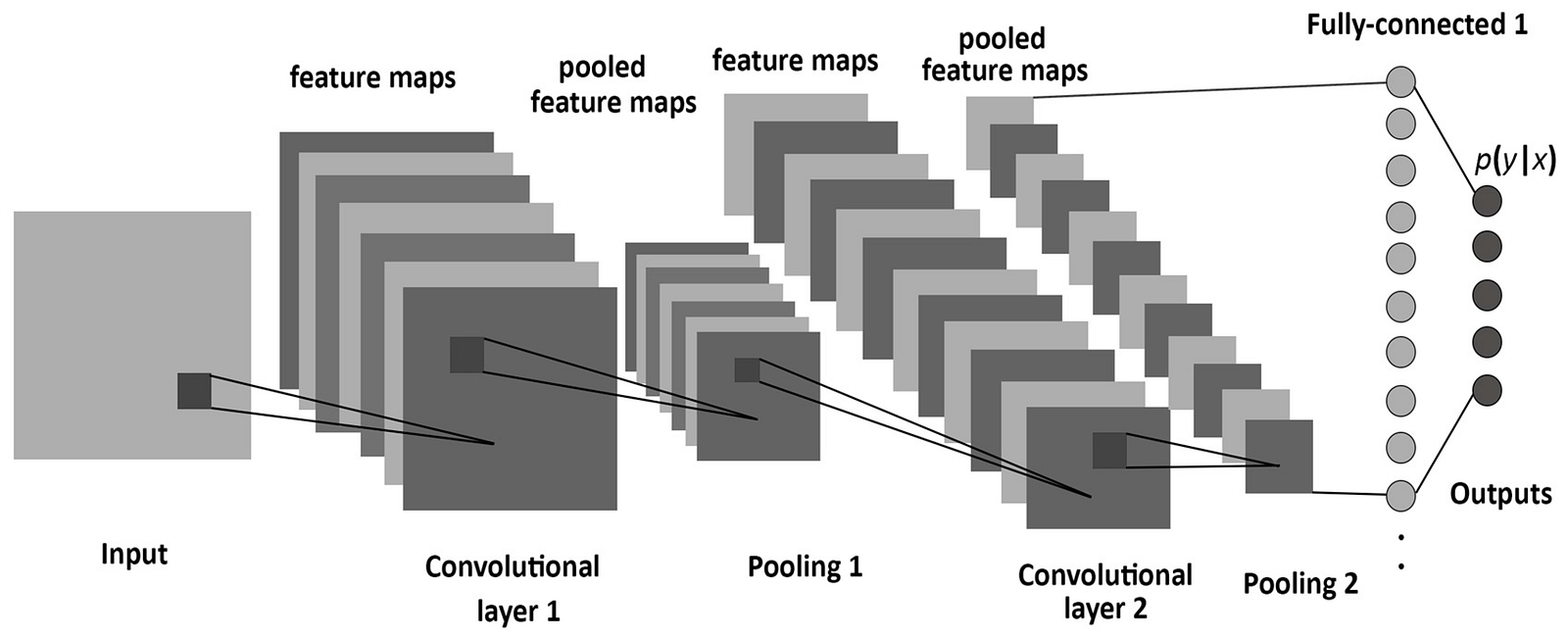To understand it in detail one needs to understand what convolution and pooling are. Both of these concepts are borrowed from the field of Computer Vision and are defined below.

Convolution: Convolution operates on two signals (in 1D) or two images (in 2D): you can think of one as the “input” signal (or image), and the other (called the kernel) as a “filter” on the input image, producing an output image (so convolution takes two images as input and produces a third as output). 

In layman terms it takes in an input signal and applies a filter over it, essentially multiplies the input signal with the kernel to get the modified signal. Mathematically, a convolution of two functions f and g is defined as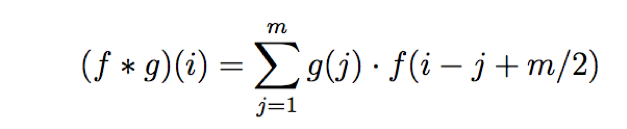which, is nothing but dot product of the input function and a kernel function.

In case of Image processing, it is easier to visualize a kernel as sliding over an entire image and thus changing the value of each pixel in the process.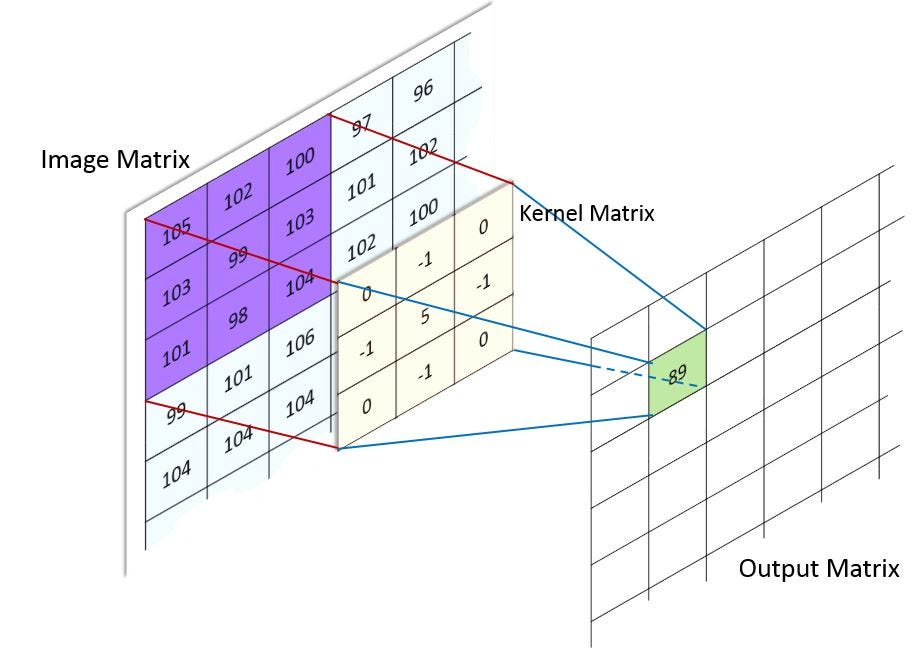Image Credit: Machine Learning Guru 

Pooling: Pooling is a sample-based discretization process. The objective is to down-sample an input representation (image, hidden-layer output matrix, etc.), reducing its dimensionality and allowing for assumptions to be made about features contained in the sub-regions binned.

There are 2 main types of pooling commonly known as max and min pooling. As the name suggests max pooling is based on picking up the maximum value from the selected region and min pooling is based on picking up the minimum value from the selected region.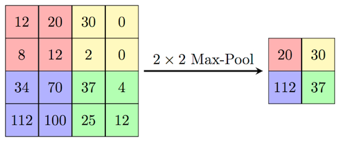Thus as one can see A Convolutional Neural Network or CNN is basically a deep neural network which consists of hidden layers having convolution and pooling functions in addition to the activation function for introducing non-linearity.

A more detailed explanation can be found at

http://colah.github.io/posts/2014-07-Conv-Nets-Modular/

Recurrent Neural Networks (RNN)

Recurrent Neural Networks or RNN as they are called in short, are a very important variant of neural networks heavily used in Natural Language Processing. In a general neural network, an input is processed through a number of layers and an output is produced, with an assumption that two successive inputs are independent of each other.

This assumption is however not true in a number of real-life scenarios. For instance, if one wants to predict the price of a stock at a given time or wants to predict the next word in a sequence it is imperative that dependence on previous observations is considered.

RNNs are called recurrent because they perform the same task for every element of a sequence, with the output being depended on the previous computations. Another way to think about RNNs is that they have a “memory” which captures information about what has been calculated so far. In theory, RNNs can make use of information in arbitrarily long sequences, but in practice, they are limited to looking back only a few steps. 

Architecture wise, an RNN looks like this. One can imagine it as a multilayer neural network with each layer representing the observations at a certain time t.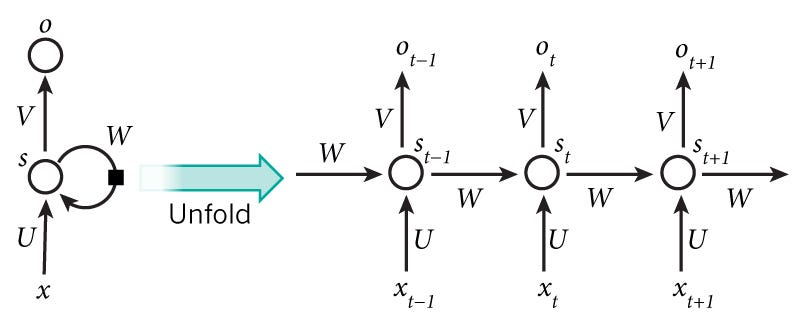RNN has shown to be hugely successful in natural language processing especially with their variant LSTM, which are able to look back longer than RNN. If you are interested in understanding LSTM, I would certainly encourage you to visit

http://colah.github.io/posts/2015-08-Understanding-LSTMs/

In this article I have tried to cover neural networks from a theoretical standpoint, starting from the most basic structure, a neuron and covering up to the most popular versions of neural networks. The aim of this write up was to make readers understand how a neural network is built from scratch, which all fields it is used and what are its most successful variations.

I understand that there are many other popular versions which I will try to cover in subsequent posts. Please feel free to suggest a topic if you want it to be covered earlier.